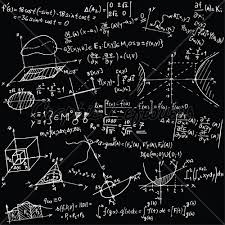# Equation 7848

Solve the equation with the unknown a: 3 (2a + 1) = (a-3) • 4-5

a =  -10

### Step-by-step explanation:

3 (2a+1) = (a-3) · 4-5

3·(2·a+1) = (a-3) · 4-5

2a = -20

a = -20/2 = -10

a = -10

Our simple equation calculator calculates it.Did you find an error or inaccuracy? Feel free to write us. Thank you!

Tips for related online calculators
Do you have a linear equation or system of equations and looking for its solution? Or do you have a quadratic equation?